# Image Compression Using Principal Component Analysis (PCA)

As data collection continues to increase daily, every organization requires efficient solutions to store and transmit data, particularly image data. The bulky nature of such data often requires significant bandwidth, making it challenging to manage.

So, there is a pressing need to develop innovative technologies capable of retaining the same data with minimal memory usage while preserving crucial information. The best thing is that we can solve such problems using machine learning. In this blog, we will build our image data compressor using an unsupervised learning technique called Principal Component Analysis (PCA).

These are some of the topics that we will explore in detail:

• Image types and quantization
• PCA overview
• Step-by-step Python implementation of PCA for image compression, resulting in an 82% reduction in size by compromising only ~2% of the information.

Let’s begin with the basics of image data first.

### Image Types

We are probably familiar with the fact that images sent through personalized chat platforms like Whatsapp are automatically reduced in size. This is to save internet bandwidth and storage space. Some websites even offer to produce the same image with a smaller file size, like the one shown below.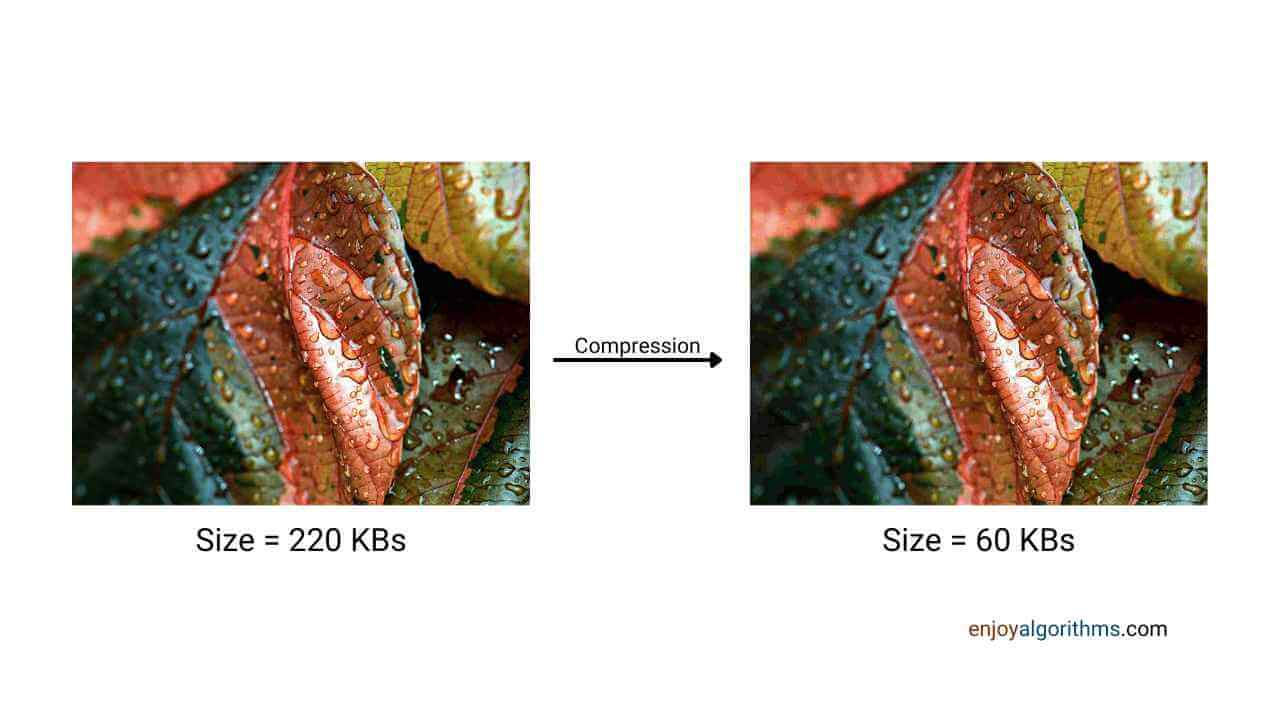But how exactly they do that? We will explore this question in greater detail. But let's first see how computers read the image data.

Computers read an image as a matrix with elements as pixel values that represent the color. 0 represents black and 255 means white. This matrix contains three dimensions, (Height x Width x Channels) or (Channels x Height x Width). Based on the number of channels present in this matrix, we can classify images into two types:

1. Grayscale image
2. RGB image

A grayscale image can be depicted in a matrix with pixel values of just one channel (Height x Width x 1), while a colored image is usually a matrix of three stacked RGB channels (Red, Green, and Blue) (Height x Width x 3).

### Quantization of ImagesThe above figure shows the 13 properly distinguishable shades of grayscale. While our eyes can easily distinguish between thousands of colors, they can hardly detect only a dozen shades of color. Each of the 13 shades can be further divided into multiple different shades. With the incorporation of more shades, the clarity between two neighboring shades diminishes. This is the fundamental property that is exploited while performing image compression.

In general, 8 bits represent the shades of an image (0 to 2^8 -1) where 0 represents complete black and 255 represents complete white. Projecting some bits to a nearby threshold makes no difference to the eye in terms of quality.

To understand it better, suppose we replace the odd pixel values (1, 3, 249, etc.) with the next even pixel value (2, 4, 250, etc.). The change in the image will not be visible or distinguishable, but we reduce the memory required to store those values. This is called quantization and is one of the simplest ways of performing image compression.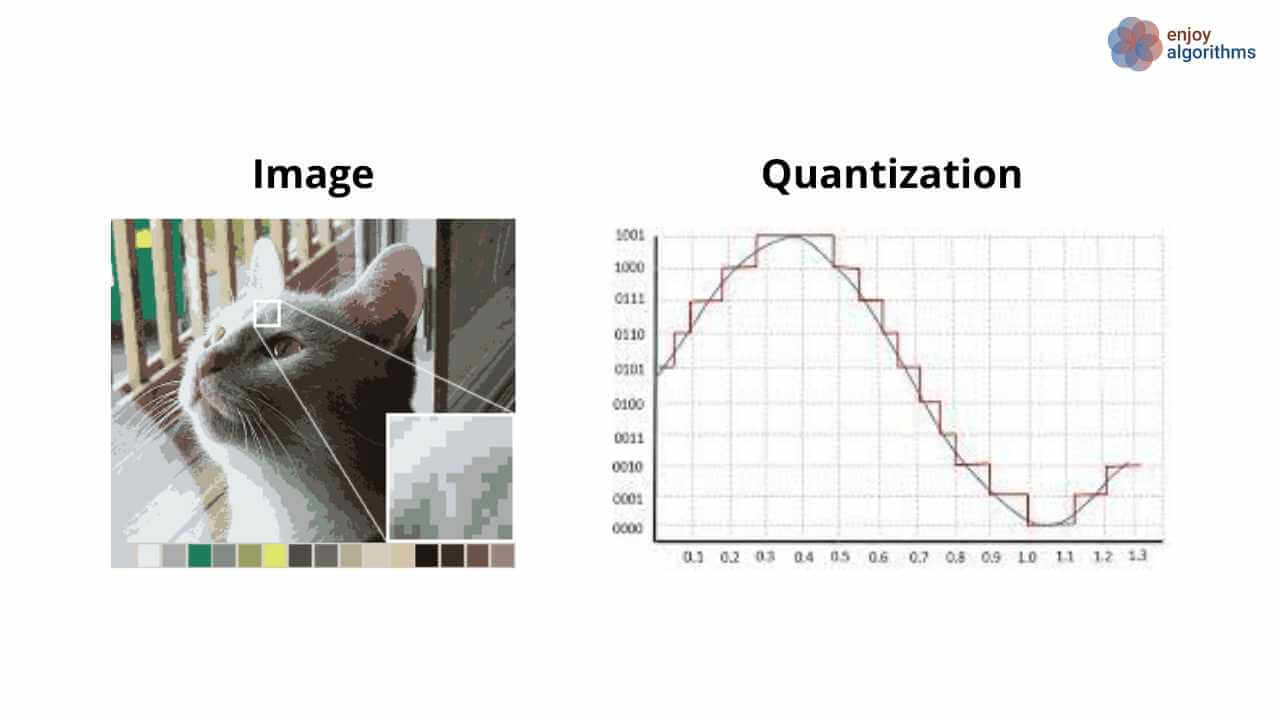However, we will use Principal Component Analysis (PCA) to compress the image. PCA-based compression works on the same principle as quantization. Still, instead of projecting the pixel bits on a certain threshold, we will be projecting them along the principal components. This project demands a basic understanding of PCA, so we recommend you see our** PCA** blog first.

### PCA Overview

PCA is an unsupervised dimensionality reduction technique where we try to transform the existing features into new features such that

1. Every feature is perpendicular to each other.
2. Features are arranged in decreasing order of information they carry.

For example, suppose we have ten features in a dataset and we are using PCA. In that case, we can create (let’s say) a five-feature dataset that can retain maximum information from the original ten features.

#### What is the purpose of this project?

The purpose of image compression is to reduce the size of the image such that the transmission consumes lesser internet bandwidth. A picture is also a signal transmitted from one place to another. As there is limited bandwidth, we don’t want to send the entire image with 100% information but the compressed version that contains as much information as possible. People would reconstruct the same image (say, 512x512) at the receiver’s end with the transmitted data.

### Steps for PCA used for RGB Image Compression

These are the steps involved in PCA-based image compression:

1. The computer will read our image as a matrix of shape height*width*channel. For grayscale images, the value of channels would be 1. If the image is in RGB format with a resolution of 1024, the matrix shape will be 1024*1024*3. We will have to consider each channel separately to perform PCA for RGB images.
2. For every channel, the shape of the matrix would be 1024*1024. We need to compute the mean and covariance of this matrix. With this, we can calculate the Eigen values and Eigen vectors. Later, these values and vectors are arranged into a decreasing order because eigenvectors corresponding to the highest eigenvalue will contain the most information.
3. Please select the first few components for the compression and remove the rest, as they do not provide much information.
4. After that, we can project the processed data along the selected components and add the mean of the image into it to get the final compressed version of our original image.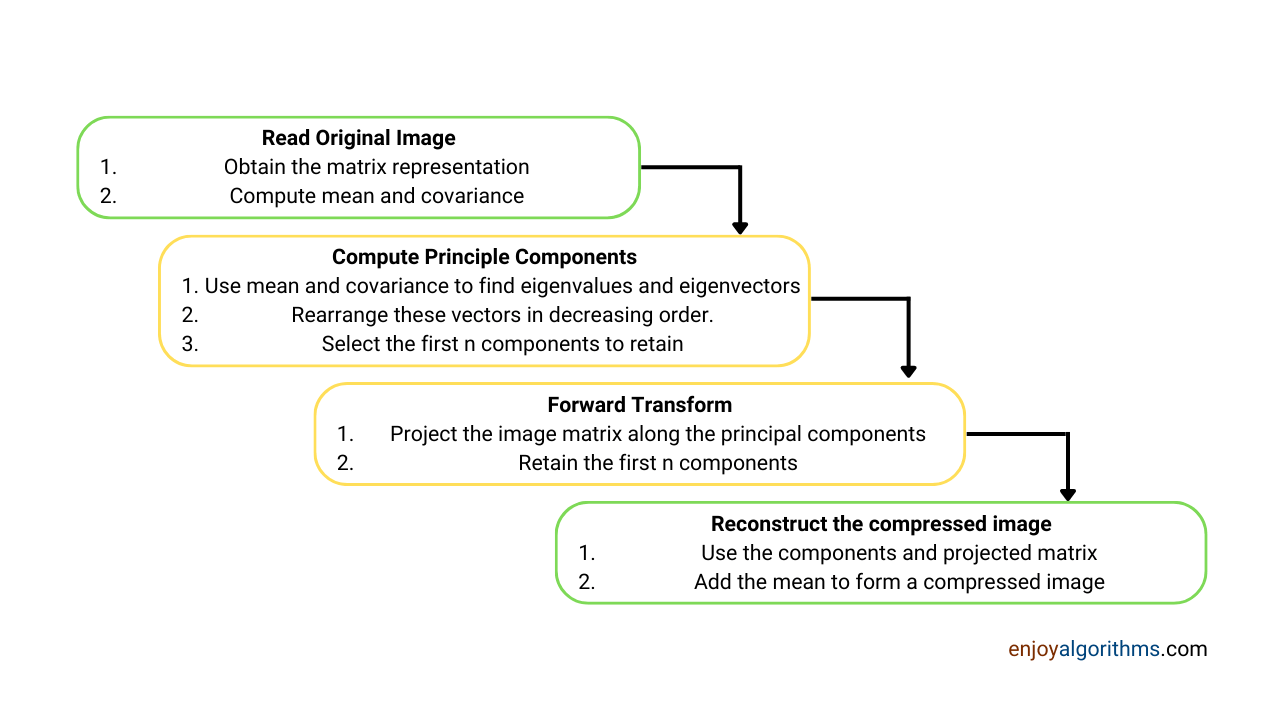The image is reconstructed from the dominant eigenvectors formed using the Principal Component Analysis in the above pipeline.

Now it's time to build the image compression project end-to-end.

### Color Image Compression Using PCA In Python

#### Step 1:Import the necessary libraries in Python

Here, we will import four basic libraries required for this application:

• PIL (Python Image Library): It enables the reading and manipulation of the image file.
• NumPy: It enables mathematical computations and manipulations of images (arrays/matrices).
• PCA: We will use PCA from the Scikit-learn framework to make our process faster. PCA is built under sklearn.decomposition
• Matplotlib: It is used for the visualization of various plots.
``````from PIL import Image, ImageOps               # Image Manipulation
from sklearn.decomposition import PCA
import numpy as np
import os
import matplotlib.pyplot as plt               # Visualization``````

#### Step 2: Reading the original image

Let's take a sample image of a random girl who does not exist. Yes! There is an AI-based website thispersondoesnotexist.com that generates a random face of a person who does not live in the real world. One can choose any other image from the mentioned website.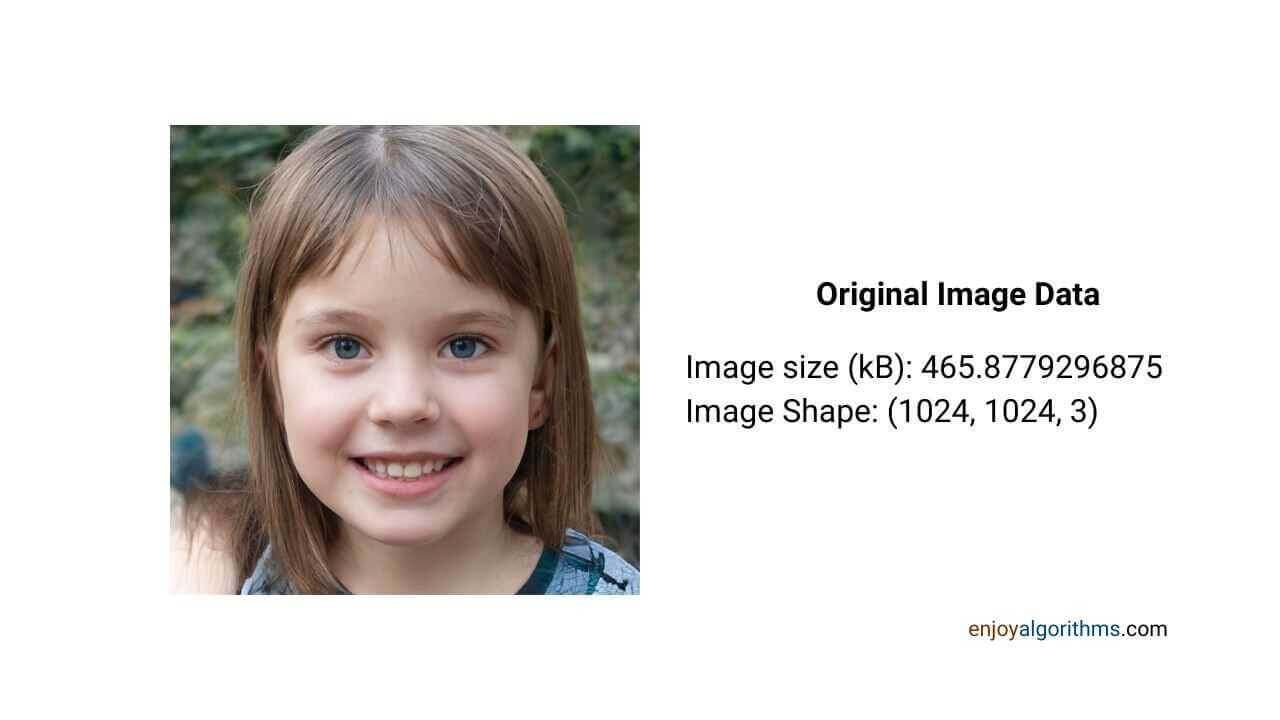We need to read our image and extract some critical properties from it. For that, we can use the Image function from the PIL library.

``````def img_data(imgPath,disp=True):

orig_img = Image.open(imgPath)

img_size_kb = os.stat(imgPath).st_size/1024
data = orig_img.getdata()
ori_pixels=np.array(data).reshape(*orig_img.size, -1)
img_dim = ori_pixels.shape

if disp:
plt.imshow(orig_img)
plt.show()

data_dict = {}
data_dict['img_size_kb'] = img_size_kb
data_dict['img_dim'] = img_dim

return data_dict``````

The above function takes in the image file's location as input and returns a python dictionary containing image properties, such as the size and dimension of the image. In our case, the image is an RGB image with dimensions 1024*1024*3, and the size is around 466 KBs.

Our Image compression project aims to reduce the file size (in KB) while keeping the overall dimensions the same as (1024*1024). Let's compute the components.

#### Step 3: Computing the principal components for the input image

The input image has three channels, i.e., Red, Green, and Blue. In this step, we will have to decompose the image into separate channels and then fit the PCA algorithm on each channel.

``````def pca_compose(imgPath):

orig_img = Image.open(imgPath)
orig_img = Image.open(imgPath)

# 2. Convert the reading into a 2D numpy array
img = np.array(orig_img.getdata())

# 3. Reshape 2D to 3D array
# The asterisk (*) operator helps in unpacking the sequence/collection as positional arguments.
# So, instead of using indices of elements separately, we can use * and perform action on it.
# print(orig_img.size) = (1024, 1024) --> print(*orig_img.size) = 1024 1024
img = img.reshape(*orig_img.size, -1)

# Seperate channels from image and use PCA on each channel
pca_channel = {}
img_t = np.transpose(img) # transposing the image

for i in range(img.shape[-1]):    # For each RGB channel compute the PCA

per_channel = img_t[i] # It will be in a shape (1,1024,1024)

# Converting (1, 1024, 1024) to (1024, 1024)
channel = img_t[i].reshape(*img.shape[:-1])  # obtain channel

pca = PCA(random_state = 42)                #initialize PCA

fit_pca = pca.fit_transform(channel)        #fit PCA

pca_channel[i] = (pca,fit_pca)  #save PCA models for each channel

return pca_channel``````

In the above function, we take image location as the input and then convert the image data into a numpy array. All the channels in that array (RGB) are individually fitted in different instances of PCA. The models and the transformed data are saved and returned in a dictionary format. Please note that for an image of size 1024*1024, we will have 1024 components as PCA transforms the existing features into the same number of new features arranged in descending order of importance.

Now, we have fit the PCA model corresponding to each channel. Let's understand how we will reconstruct the final compressed image.

#### Step 4: Lossless Reconstruction of the image using a reduced number of components

In this stage, the saved PCA models for each channel obtained in the previous step are used for reconstruction. The variable n_components decides how many of the top 1024 principal components will be retained for the reconstruction. One good observation can be made that if we retain all 1024 components, there won't be any size reduction. Our objective is to reduce the size of the image at the cost of very slight information loss. In the case of an image, we can sense that information from the clarity of the output image.

``````# Function to select the desired number of components
def pca_transform(pca_channel, n_components):

temp_res = []

# Looping over all the channels we created from pca_compose function

for channel in range(len(pca_channel)):

pca, fit_pca = pca_channel[channel]

# Selecting image pixels across first n components
pca_pixel = fit_pca[:, :n_components]

# First n-components
pca_comp = pca.components_[:n_components, :]

# Projecting the selected pixels along the desired n-components (De-standardization)
compressed_pixel = np.dot(pca_pixel, pca_comp) + pca.mean_

# Stacking channels corresponding to Red Green and Blue
temp_res.append(compressed_pixel)

# transforming (channel, width, height) to (height, width, channel)
compressed_image = np.transpose(temp_res)

# Forming the compressed image
compressed_image = np.array(compressed_image,dtype=np.uint8)

return compressed_image``````

The transformed data is projected onto the chosen eigenvectors, and the mean is added to obtain the final compressed image. We can choose to retain around 5%of 1024(~= 50) components for the original image and project the data on the same.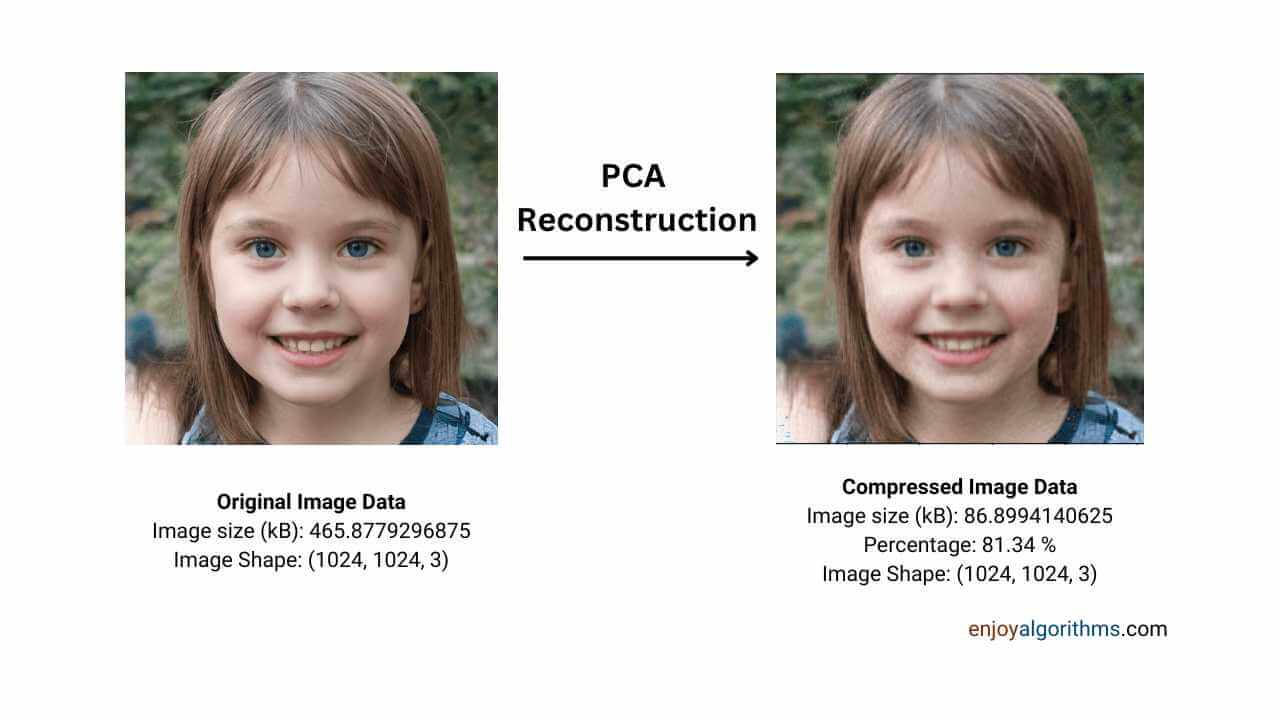When we are using just 5% of the principal components, a reduction of ~380KB (81.34%) was obtained while retaining 97.778% of the variance/information of the original image. But one interesting question to ask is,

#### How do we decide the value of n_components?

Let's understand the technicalities behind how we can find the number of components we need to retain not to lose much information from the final image. For that, we first need to know which component carries the percentage of information with it. As we already know, PCA arranges the components in decreasing order. Still, the number of components needs to be retained to safe keep most information easily if we know the exact percentage contained by individual components.

#### Variance Explained vs. Principal Components

The function below tells us how much information will be retained by selecting n number of components. This n_components is an input parameter of the function, and we can vary it to observe the changes.

``````# Function to tell the percentage of explained variance by n number of components
def explained_var_n(pca_channel, n_components):

var_exp_channel = []; var_exp=0

for channel in pca_channel:

pca,_ = pca_channel[channel]

var_exp_channel.append(np.cumsum(pca.explained_variance_ratio_))

var_exp += var_exp_channel[channel][n_components]

var_exp = var_exp/len(pca_channel)

return var_exp``````

In the image below, the first image shows the individual information percentage carried by every principal component, and the second image shows the aggregate of the information held by the first n_components.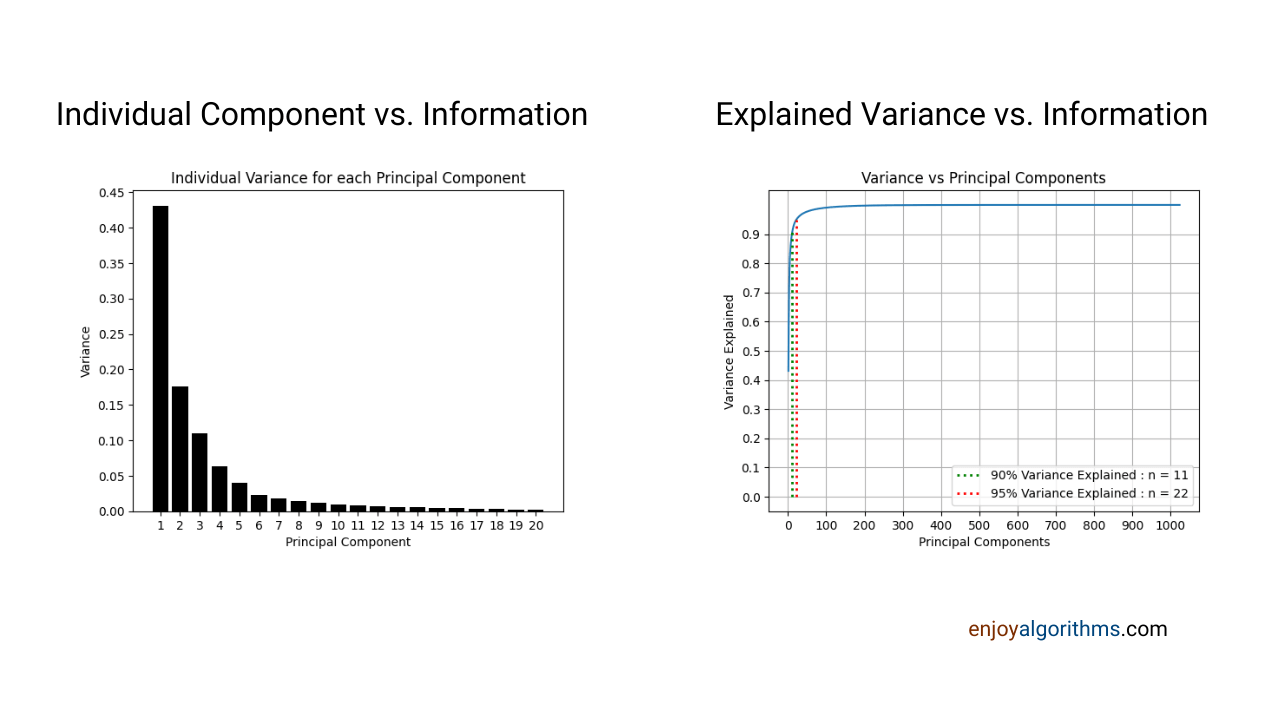One can see that the first principal component retained ~43% of the total variance. As we move to the 20th principal component, less than 1% of the total variance was owned by that component. We can observe that the cumulative retention of the variance is directly proportional to the number of principal components taken. In simple terms, if we keep increasing the number of components, the more information we will retain, resulting in a poor compression percentage.

Similarly, we can plot the percentage reduction in the size of the compressed image (KBs) vs. the number of components we will retain.

#### File Size (kB) vs. Principal Components

The percentage reduction in size is inversely proportional to the number of principal components used. We should use the minimum number of principal components if we want more reduction.

In our example, we can reduce the image to 50% of its original by selecting only six principal components. We need at least 25 principal components to retain 96% of the variance of the original image. There is a tradeoff between retaining more information and better compression in size. We should always keep an optimum number of components that balance the explained variability and the image quality with the desired size.

One can find the complete code in EnjoyAlgorithm's Machine Learning GitHub repository.

### Possible Interview Questions on Image Compression Using PCA

In case readers are planning to put this project into their resumes, these are the possible questions the interviewer can ask:

• Question: What is the basic principle used in Image Compression?
Answer: Quantization is the most fundamental principle used to perform image compression.
• Question: What is Image Quantization?
Answer: We project/replace the image pixel values with a particular threshold value in quantization.
• Question: Can we perform compression without losing any information?
Answer: The shortest answer would be No because, in compression, we try to solve the tradeoff between Compressed image size and the number of components retained.
• Question: How do we decide the number of components required to achieve the compression?
Answer: PCA arranges the components in a descending order w.r.t the information they carry. If we progressively keep increasing the number of components and simultaneously observe the percentage reduction, we can stop at some optimum value.
• Question: Why do we add the mean of the image for reconstruction?
Answer: The input data is getting standardized before fitting PCA. Hence at the time of reconstruction, we bring the image pixels into the same space by adding mean.

### Conclusion

In this complete machine learning project blog, we used an unsupervised learning approach, PCA, to reduce the size of the image. We learned how to decide the number of components to keep and simultaneously focused on the part where we could retain as much information as possible. We also discussed the reconstruction of the images after performing PCA. As a bonus, we presented the complete code of this implementation along with the GitHub link. We hope you find the article enjoyable and informative.

If you have any queries/doubts/feedback, please write us at contact@enjoyalgorithms.com. Enjoy learning, Enjoy algorithms!

☆ 16-Week Live DSA Course
☆ 10-Week Live DSA Course